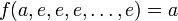# Neutral element for a multiary operation

Jump to: navigation, search

## Definition

### All-sided neutral element

Suppose$S$ is a set and$f:S^n \to S$ is a$n$-ary operation for$f$. An element$e \in S$ is termed a neutral element for$f$ if the following holds:$f$ evaluated at any$n$-tuple where$n - 1$ of the entries are equal to$e$ and the remaining entry is$a \in S$, gives output$a$. This is true regardless of where we place$a$ and is also true if$a = e$.

The term neutral element, when used without qualification, is used in the context$n = 2$, i.e., for a binary operation, i.e., a magma.

### Left neutral element

Suppose$S$ is a set and$f:S^n \to S$ is a$n$-ary operation for$f$. An element$e \in S$ is termed a left neutral element for$f$ if the following holds:$f(e,e,e,\dots,e,a) = a$ for all$a \in S$.

### Right neutral element

Suppose$S$ is a set and$f:S^n \to S$ is a$n$-ary operation for$f$. An element$e \in S$ is termed a right neutral element for$f$ if the following holds:$f(a,e,e,e,\dots,e) = a$ for all$a \in S$.

### Neutral element for a given position

Suppose$S$ is a set and$f:S^n \to S$ is a$n$-ary operation for$f$. Suppose$i \in \{ 1,2,\dots,n \}$. An element$e \in S$ is termed a neutral element for position$i$ if$f$ if the following holds:$f(e,\dots,e,a,e,e,\dots,e) = a$ for all$a \in S$ where$a$ appears in the$i^{th}$ position.

## Facts

The case$n = 2$ is special because we can deduce equality of left and right neutral element and therefore also deduce that binary operation on magma determines neutral element. For higher$n$, there could be more than one neutral element.